## Interest-Only Loan Part 2: Calculating the Paydown Payment

Categories:

The basics on calculating the paydown payment on the amortization part of an interest-only loan.

There are two parts to an interest-only loan. Part one is just interest, and part two is the paydown, or amortization.

You never have to wait to get to the paydown in order to refinance your interest-only loan. Some investors refinance the same interest-only property over and over before ever getting to the paydown part.

But it’s important to know the numbers even if you don’t want to keep an interest-only loan until the paydown. Here are the basics of calculating 30-year and 40-year interest only loans.

## Calculating The Paydown

The interest-only portion of an interest-only loan lasts for a set number of years. For example, let’s say ours lasts 10 years.

The paydown period is when the loan starts amortizing – the actual amount borrowed starts going down. However, you’ll still need to pay normal interest along with the principal payment.

With most lenders, you’ll get either a 30-year or 40-year loan. A 30-year interest-only loan would involve 10 years of just interest, plus 20 years of paydown. For a 40-year, you’d have 30 years’ worth of amortization payments.

A 30-year loan’s payments will be higher because you’re paying the same amount off in a shorter period of time.

## Calculating a Paydown Payment Example

Let’s break down the difference between a 30-year and 40-year interest-only loan.

30-year loan = 10 years interest, then 20 years of amortization

40-year loan = 10 years interest, then 30 years amortization

You can use an amortization calculator tool to figure your monthly payments for the paydown period.

Let’s look at an example for a \$300,000 interest-only loan. The paydown period payments would be:

30-year =  10 years of \$2,000/month + 20 years of \$2,509/month

40-year =  10 years of \$2,000/month + 30 years of \$2,201/month

Remember that you’re never locked into paying a full interest-only loan. An interest-only loan may be worth looking into for your property. Especially if you need a product with lower monthly payments while you wait out rising interest rates.

## Help with Interest-Only Loans

Have questions about interest-only loans, or calculating your paydown payment? Is there a deal you’d like us to take a look at?

We search hundreds of loans every month – now is a great time of variety in loan products. We’d love to help you find exactly what you need.

Email us at Info@TheCashFlowCompany.com.

Watch the video here:

## How to Calculate a DSCR Loan – Step By Step

Categories:

From figuring out expenses to doing the math… Here’s how to calculate a DSCR loan.

The more you know about DSCRs, the more power you have when you go to buy or refinance.

When you practice the numbers yourself, the less you have to rely on a lender to tell you if a deal is good or not.

## What Is a DSCR?

DSCR means debt service coverage ratio. It’s a loan for rental properties that hinges on cash flow.

A DSCR loan will be a useful product in your real estate investing career. It requires no income verification and no work or investment history. These loans only require that the property’s income is the same (or higher than) the expenses.

Cash flow is always important to you as an investor, and for DSCR loans, it matters just as much to your lender. The better your cash flow, the better LTV and rates you can get.

But even if your property has negative cash flow, you still can get DSCR loans. You’ll just have to pay for it when it comes to the LTV and interest rate.

## Calculate a DSCR Loan Expenses

You can follow along with your DSCR loan calculator (free download here). We’ll fill out these form to show each step of how to calculate a DSCR loan.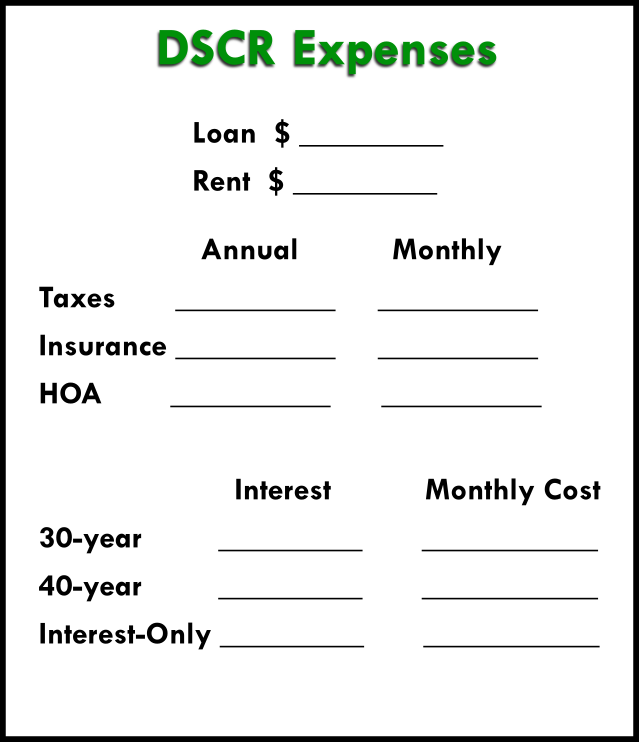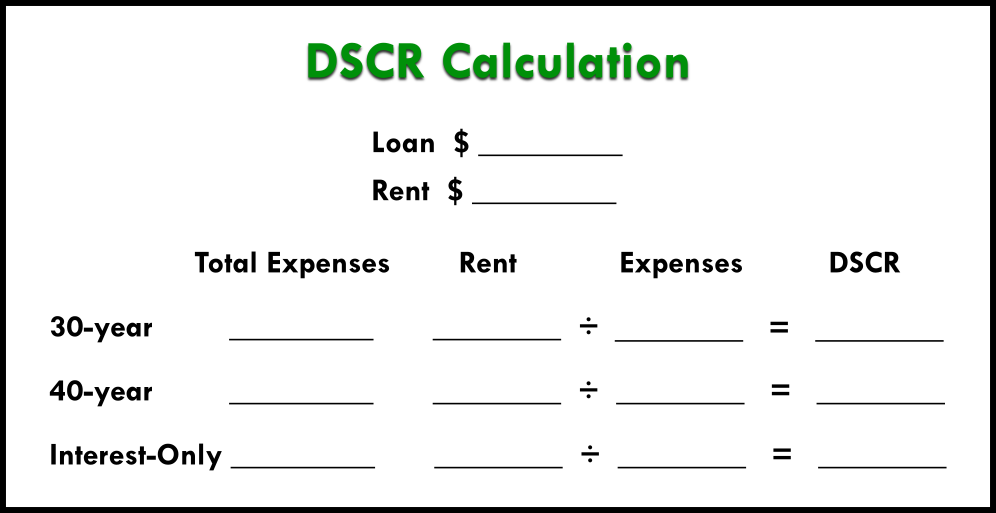### Rent Income & Loan Amount

Firstly, you need to estimate your loan amount and your rent income. If you have a deal in front of you, you probably have a good idea of the loan amount you’ll need to be able to afford the property.

As for rent, you can get realistic amounts from online sources. Look at Zillow or Rent.com to find the market rate for rent in the property’s neighborhood.

Let’s keep it simple for our example and say our loan is \$200,000, and our rent income is \$2,000.

### What Expenses Count in a DSCR Loan?

We know our income (rent), but now we need to figure out our costs.

The expenses considered in a DSCR loan DO include:

Taxes

Insurance

HOA fees

Expenses NOT considered in a DSCR loan are things like:

Property management fees

Utilities

Maintenance

To estimate the taxes on the property, you could use a property tax calculator like this one. If you need an estimate on insurance, you can try this home insurance calculator. You can figure out HOA fees by contacting the HOA, if that applies to your property.

If any of these costs are charged annually, then you’ll need to divide by 12 to break it down into a monthly cost.

Let’s take a look at what information we have now for our example DSCR loan: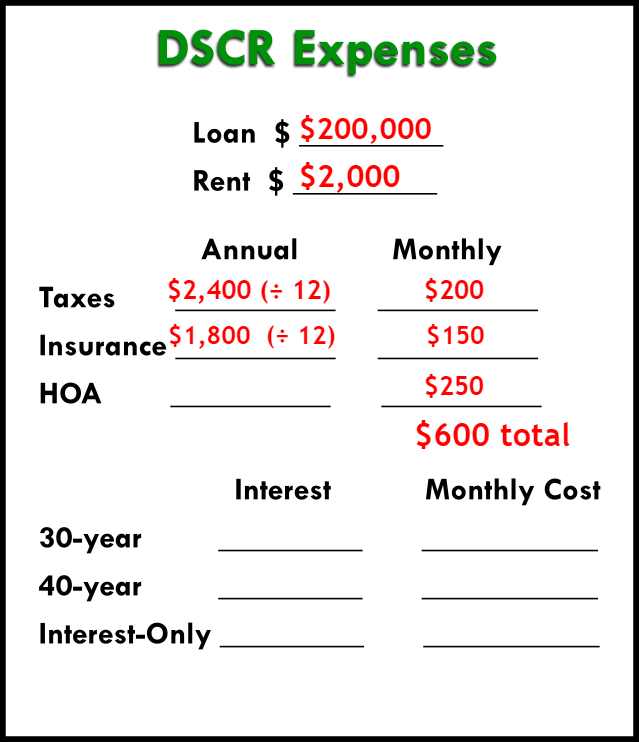### Calculating Loan Cost

Secondly, DSCR lenders will offer many types of the loans – fixed-rate mortgages, interest-only, ARMs, etc. You need to find what best fits you, and to do that, you’ll have to run all the numbers.

To calculate each of the amortized loans, you can use an amortization calculator like this one. Add in your information – loan amount, interest rate, and loan length.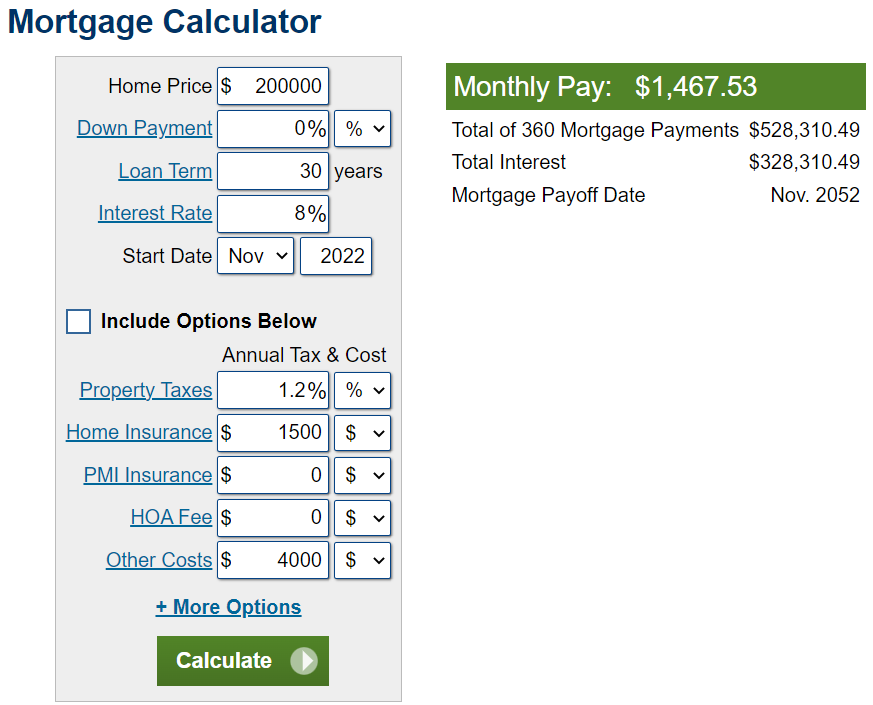We’re going to use an 8% interest rate for our example, since that’s the anticipated average for next year.

In reality, each loan and lender will have a different interest rate. Additionally, the interest rate may fluctuate depending on your qualifications and DSCR. You can get this information from your lenders to plug into your calculator.

We’ll use three common loans for this example: a 30-year fixed, 40-year fixed, and interest only loan.

If we had a 30-year mortgage for \$200,000 at 8%, our monthly payment would be \$1,467.

For a 40-year fixed with the same info, payments would be \$1,390.

For interest-only, you can calculate the loan fairly simply yourself. Multiply the loan amount by the interest rate (e.g., 200,000 × .08 = 16,000). That gives you the yearly interest, then you divide it by 12 to get the monthly payment. For our example, that’s \$1,333.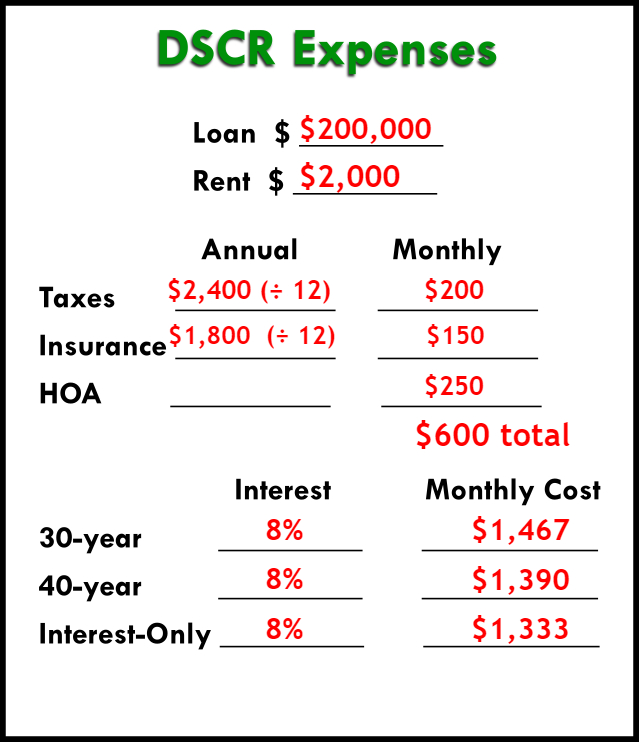So what do you do with these numbers? How do you know which loan is best?

It depends on your priorities. To have the most cash flow, the lowest number is best (in this case, interest-only). If you need something that amortizes, a 30-year would probably be best.

But you don’t really know which loan will be best for you until you calculate the DSCR.

## How to Calculate the DSCR

Loan LTVs and rates on a DSCR are determined by the debt service coverage ratio itself. Now that we have all our raw information, we can plug it into our DSCR calculation to get the ratio.

Here’s how you get the numbers you need:

Add up your expenses (taxes, insurance, and HOA fees) with each loan’s payment amount. Then divide rent by all those expenses.

Costs + Mortgage = Total Expenses

Rent ÷ Total Expenses = DSCR Ratio

Here’s an example of what it would look like with an example using a \$200,000 loan and an 8% interest rate: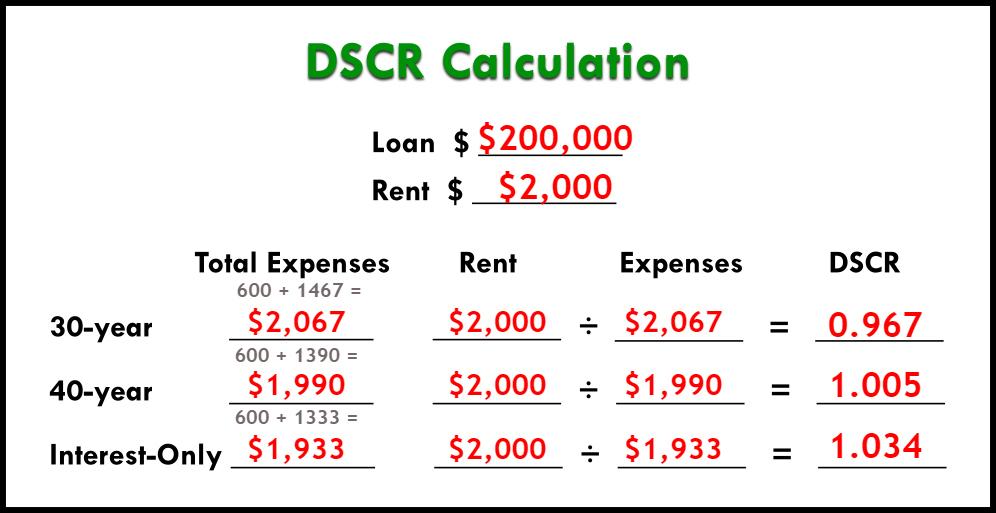We want the DSCR to at least equal 1.

Over 1 is ideal. This is a higher cash flow, and you’ll get a better loan.

Less than 1 means negative cash flow, and means you might have to look at a negative DSCR or a no-ratio loan instead.

<1 = Negative Cash Flow

At 1 = Rent = Expenses

>1 = Positive cash flow

## Help with How to Calculate a DSCR Loan

Do you have a deal with a DSCR of 1 or more? Do you need help finding out?

If you have any questions, we’d be glad to help. If you have a deal, we can run the numbers for you. Email us at Info@TheCashFlowCompany.com.

You have choices in the DSCR world. Let us help you find them!